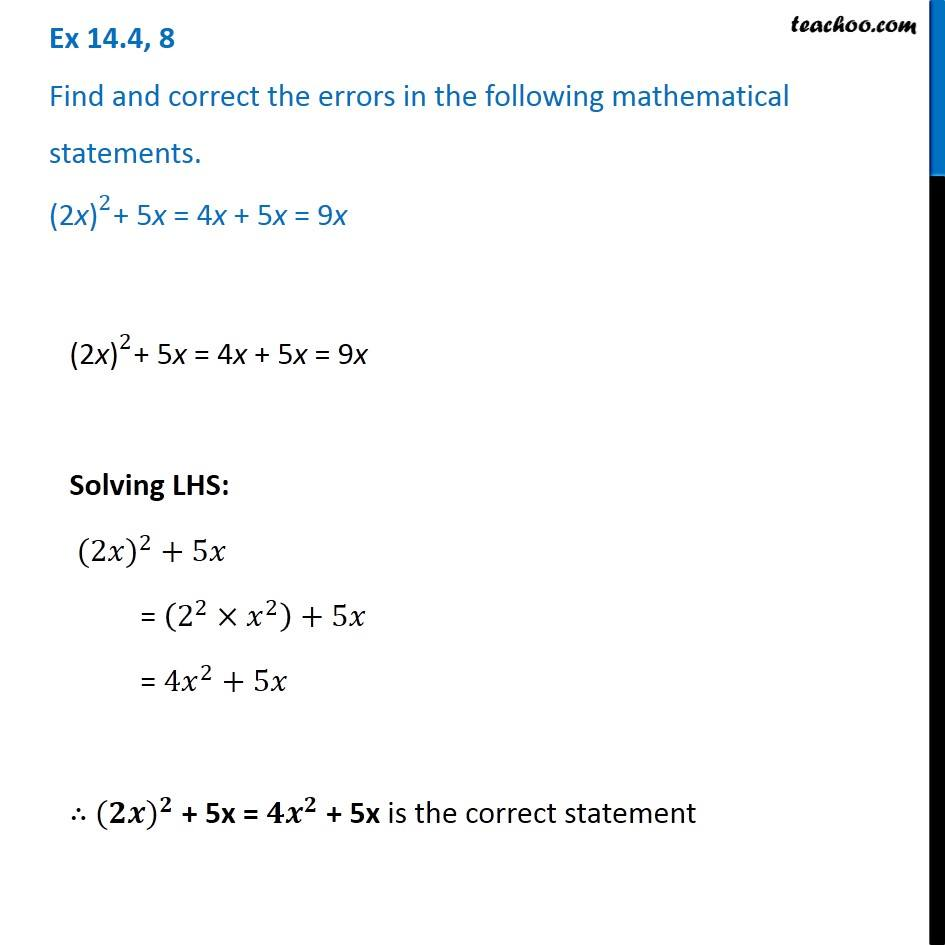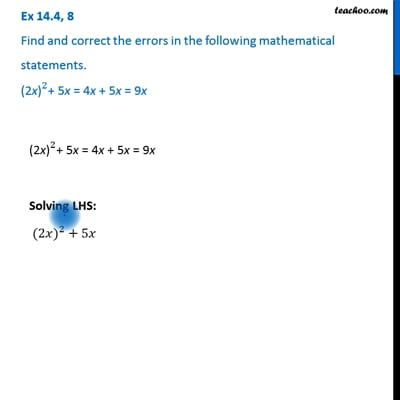Ex 14.4

Chapter 14 Class 8 Factorisation
Serial order wiseThis video is only available for Teachoo black users

Get live Maths 1-on-1 Classs - Class 6 to 12

### Transcript

Ex 14.4, 8 Find and correct the errors in the following mathematical statements. 〖"(2x)" 〗^2+ 5x = 4x + 5x = 9x 〖"(2x)" 〗^2+ 5x = 4x + 5x = 9x Solving LHS: 〖(2𝑥)〗^2 + 5𝑥 = (2^2×𝑥^2 )+5𝑥 = 〖4𝑥〗^2+5𝑥 ∴ 〖(𝟐𝒙)〗^𝟐 + 5x = 〖𝟒𝒙〗^𝟐 + 5x is the correct statement